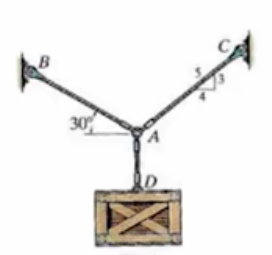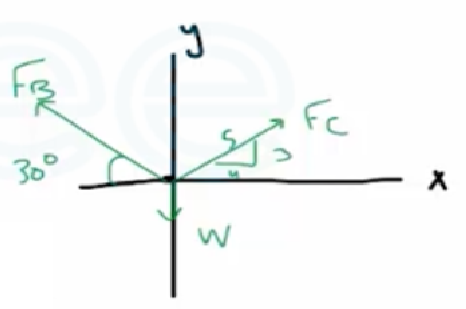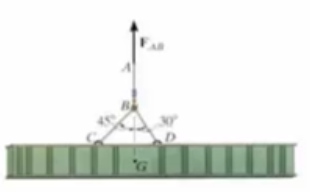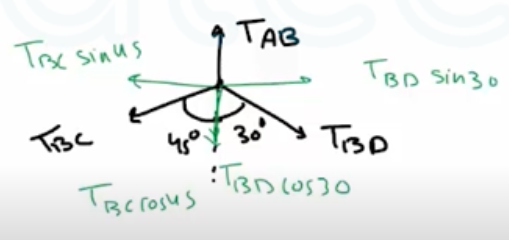Need Help?

Subscribe to Statics

###### \${selected_topic_name}
• Notes

The crate has a weight of  $2.75 \mathrm{kN}$ . Determine the  force in each supporting cable.$\stackrel{+}{\rightarrow} \sum F_{x}=0$

$F_{c}\left(\frac{4}{5}\right)-F_{B} \cos 30=0 \leftarrow$

$+\uparrow \sum F_{y}=0$

$F_{B} \sin 30+F_{c}\left(\frac{3}{5}\right)-2.75=0$

$0.5 \mathrm F_{B}+\left(1.08 \mathrm{F}_{B}\right)(0.6)=2.75\longleftarrow(2)$

$∴ F_{B}=2.395 \mathrm{kN}$

$∴ F_{c}=2.59 \mathrm{kN}$

If the mass of the girder is  $3 \mathrm{Mg}$ and its center of mass is located at point  $G,$ determine the tension developed incables  $A B,$ $B C,$ and  $B D$  for equilibrium.$\uparrow \mathrm{F}_{\mathrm{AB}}$

$\downarrow T_{A B}$

$+\uparrow \sum F_{y}=0 \rightarrow F_{A B}-T_{A B}=0$

$∴ T_{A B}=F_{A B}=W$

$w=\left(3 * 10^{3}\right)*9 \cdot 81=29.43 \mathrm{kN}$

$T_{A B}=29.43 \mathrm{kN}$$\stackrel{+}{\rightarrow} \sum F_{x}=0$

$T_{BD} sin 30 - T_{BC} sin 45 = 0$

$T_{BD} =1.414 \ T_{BC}$

$+\uparrow \sum F_{y}=0\longrightarrow T_{AB}-T_{BC} cos 45-T_{BD} cos 30=0$

$29.43-0.707 \ T_{BC}-(1.414 \ T_{BC}) 0.866=0$

$∴ T_{BC}=15.24KN \longrightarrow T_{BD}=1.414 (15.24)=21.54KN$